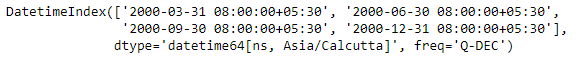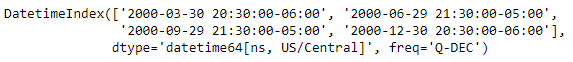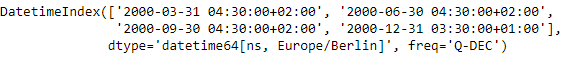# Python | Pandas DatetimeIndex.tz_convert()

• Last Updated : 24 Dec, 2018

Python is a great language for doing data analysis, primarily because of the fantastic ecosystem of data-centric python packages. Pandas is one of those packages and makes importing and analyzing data much easier.

Pandas` DatetimeIndex.tz_convert()` function convert tz-aware DatetimeIndex from one time zone to another. The function takes an input parameter which is the time zone to which we want to convert the current DatetimeIndex object to.

Syntax: DatetimeIndex.tz_convert(tz)

Parameters :
tz : Time zone for time. Corresponding timestamps would be converted to this time zone of the DatetimeIndex. A tz of None will convert to UTC and remove the timezone information.

Return : normalized : DatetimeIndex

Example #1: Use `DatetimeIndex.tz_convert()` function to convert the given DatetimeIndex object to the desired time zone.

 `# importing pandas as pd ` `import` `pandas as pd ` ` `  `# Create the DatetimeIndex ` `# Here 'Q' represents quarter end frequency  ` `didx ``=` `pd.DatetimeIndex(start ``=``'2000-01-15 08:00'``, freq ``=``'Q'``, ` `                            ``periods ``=` `4``, tz ``=``'Asia/Calcutta'``) ` ` `  `# Print the DatetimeIndex ` `print``(didx) `

Output :Now we want to convert the timezone from ‘Asia/Calcutta’ to ‘US/Central’

 `# Convert the timezone to 'US / Central' ` `didx.tz_convert(``'US/Central'``)<``/``div> `

Output :As we can see in the output, the function has changed the timezone of the didx object.

Example #2: Use `DatetimeIndex.tz_convert()` function to convert the given DatetimeIndex object to the desired time zone.

 `# importing pandas as pd ` `import` `pandas as pd ` ` `  `# Create the DatetimeIndex ` `# Here 'Q' represents quarter end frequency  ` `didx ``=` `pd.DatetimeIndex(start ``=``'2000-01-15 08:00'``, freq ``=``'Q'``,  ` `                           ``periods ``=` `4``, tz ``=``'Asia/Calcutta'``) ` ` `  `# Print the DatetimeIndex ` `print``(didx) `

Output :Now we want to convert the timezone from ‘Asia/Calcutta’ to ‘Europe/Berlin’

 `# Convert the timezone to 'Europe / Berlin' ` `didx.tz_convert(``'Europe/Berlin'``) `

Output :As we can see in the output, the function has changed the timezone of the didx object.

My Personal Notes arrow_drop_up
Recommended Articles
Page :